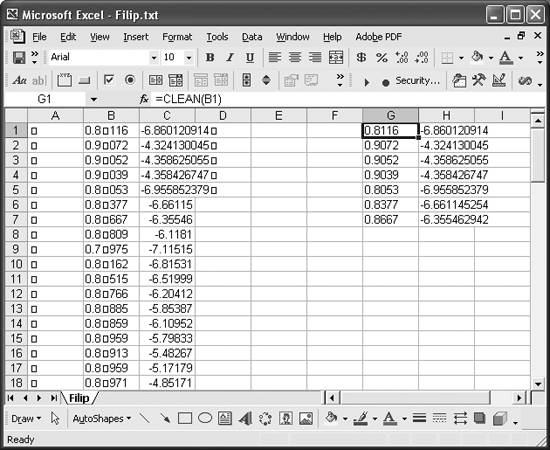# Converting Units

Problem

You want to convert a number from one unit to another directly within Excel.

Figure 3-15. CLEANSolution

Use Excel's built-in worksheet function CONVERT. Alternatively, you can write your own conversion function.

Discussion

The sections below describe hoe to use CONVERT, how to write your own conversion function (or other functions), and how to create an add-in to make your own collection of functions accessible from any spreadsheet.

Using CONVERT

The Analysis ToolPak add-in included in Excel contains a worksheet function called CONVERT that allows you to convert values from one unit to another. To use CONVERT, you must ensure the Analysis ToolPak is installed in your version of Excel. To do this, select Tools [Image_Link]/images/U2192.jpg border=0> Add-Ins ... from the main menu bar to open the Add-Ins dialog box. Select Analysis ToolPak from the list of available add-ins and then press OK.

The syntax for CONVERT is =CONVERT(cell reference, from units, to units), where cell reference is a valid cell reference, from units is a string indicating the units of the value being converted, and to units is a string indicating the units to which the value is being converted.

Valid strings for units are listed in the Excel help topic "Convert." You can find this topic by searching for "Convert" in Excel's online help, or you can browse this topic starting from the help table of contents (look for the topic Working with Data [Image_Link]/images/U2192.jpg border=0> Function Reference [Image_Link]/images/U2192.jpg border=0> Engineering Functions [Image_Link]/images/U2192.jpg border=0> Convert).

CONVERT supports a basic set of units, including length, mass, time, force, pressure, energy, power, magnetism, temperature, and volume. It also supports a range of prefixes for tera-, giga-, centi-, pica-, and so on.

You use CONVERT in a worksheet by entering the function in a cell, as illustrated in the following examples:

``` =CONVERT(A1, "in", "ft")
=CONVERT(A2, "in", "cm")
=CONVERT(A3, "HP", "MW")
```

In the first example, the value in cell A1 is converted from inches to feet. Notice how the from units and to units strings must be included in quotes. In the second example, the value in A2 is converted from inches to centimeters. In this case, the units string for meters, m, is prefixed with the centi- prefix, c, to yield the centimeters string, cm. In the third example, the value in A3 is converted from horsepower to megawatts. Here, MW is formed by prefixing the watts units string, W, with the mega- prefix, M. I should point out that these units strings are case-sensitive. For example, MW yields megawatts; however, mW yields milliwatts.

While CONVERT is a handy conversion function, it is limited to a set of very common units. You'll probably find that units common to your specialty are not included in CONVERT's lexicon. For example, in the field of naval architecture, the standard weight unit used for ships and cargo is the long ton, or LT. CONVERT does not support long tons. In fact, it does not support tons of any form.

Since converting units is a common task when pre- and post-processing data, you may find it helpful to write your own custom unit-conversion functions to supplement those supported by CONVERT. Example 3-1 shows a few custom conversion functions I use quite often.

Example 3-1. Custom unit-conversion functions

 ```Public Function LbsToLT(value As Double) As Double LbsToLT = value / 2240# End Function Public Function LbsToTons(value As Double) As Double LbsToTons = value / 2000# End Function Public Function cuFtTocuM(value As Double) As Double cuFtTocuM = value * 0.028316846592 End Function ```

You can add these or similar functions to your own spreadsheets as explained in Recipe 2.2. Be sure to put these in a new code module as explained in Chapter 2. You'll then be able to call these functions as cell formulas anywhere in your spreadsheet.

If you're like me, you'll find yourself building a custom library of useful functions and subroutines that you'd like to use repeatedly in different spreadsheets. To make your library of custom functions accessible from any spreadsheet, convert it to an add-in. Creating an add-in is easy. Here are the steps:

1. Open a workbook and add your custom functions to a code module as discussed earlier.
2. Save your workbook as an add-in. Select FileSave As ... to open the Save As dialog box. Then select "Microsoft Excel Add-in (*.xla)" from the "Save as type drop-down listbox. Doing so will automatically change the Save In folder to the default Excel add-ins folder on your machine (it's usually something like C:Documents and SettingsUserApplication DataMicrosoftAddIns). Close your add-in.
3. Open a new workbook.
4. Select ToolsAdd-Ins ... from the main menu bar to open the Add-Ins dialog box.
5. ### Sorting DataExcel Scientific and Engineering Cookbook (Cookbooks (OReilly))
ISBN: 0596008791
EAN: 2147483647
Year: N/A
Pages: 206
Authors: David M Bourg